# Abelian function

A generalization of the concept of an elliptic function of one complex variable to the case of several complex variables. A functionin the variables,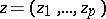, which is meromorphic in the complex space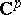,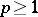, is called an Abelian function if there exist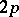row vectors in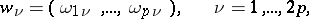which are linearly independent over the field of real numbers and are such thatfor all,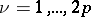. The vectors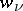are called the periods or the system of periods of the Abelian function. All periods of the Abelian function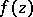form an Abelian groupunder addition, which is known as the period group (or the period module). A basis of this group is known as a basis system of periods of the Abelian function, or also as a system of basic (or primitive) periods. An Abelian functionis said to be degenerate if there exists a linear transformation of the variables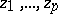which convertsinto a function of fewer variables; otherwiseis said to be a non-degenerate Abelian function. Degenerate Abelian functions are distinguished by having infinitely small periods, i.e. for any numberit is possible to find a periodfor whichIf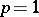, the non-degenerate Abelian functions are elliptic functions of one complex variable. Each Abelian function with period groupis naturally identified with a meromorphic function on the complex torus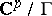, i.e. the quotient space(cf. Quasi-Abelian function).

The study of Abelian functions began in the 19th century in connection with the inversion of Abelian integrals (cf. Abelian integral) of the first kind (cf. Jacobi inversion problem, , ). The Abelian functions obtained in solving this problem are called special Abelian functions; sometimes, in earlier work, only such functions were considered to be Abelian functions. Letbe linearly independent normal Abelian integrals of the first kind, constructed on a Riemann surface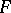: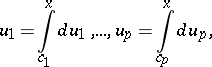and letbe a given system of sums in which the lower integration limits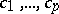are considered as fixed on the surface. It is then possible to define the special Abelian functions as all rational functions in thecoordinates of the upper limits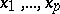, the latter being in turn considered as functions ofpointson. In the symbolic notation introduced by K. Weierstrass, any special Abelian function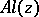may be represented as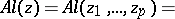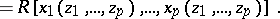The complex toricorresponding to special Abelian functions are the Jacobi varieties of algebraic curves.

The matrixwhose columns form a period basis of the Abelian functionhas dimensionand is known as the period matrix of the Abelian function. A necessary and sufficient condition for a given matrix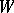of dimensionto be the period matrix of some non-degenerate Abelian functionis for the matrix to satisfy the following conditions (the Riemann–Frobenius conditions). It must be a Riemann matrix, i.e. there must exist an anti-symmetric non-degenerate square matrixwith integer elements, of order, and such that 1), where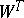is the transposed matrix of; and 2) the matrix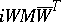defines a positive-definite Hermitian form . If the conditions 1) and 2) are expressed as equations and inequalities respectively, a system of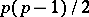Riemann equations andRiemann inequalities is obtained. The numberis called the genus of the matrixand of the corresponding Abelian function. The columns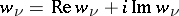of, regarded as vectors in the real Euclidean space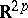, define the period parallelotope of.

All Abelian functions corresponding to the same period matrixform an Abelian function field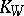. If the fieldcontains a non-degenerate Abelian function, its degree of transcendence over the field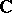is; the torusis then an Abelian variety, andturns out to be its field of rational functions. If, on the other hand, all Abelian functions ofare degenerate, thenis isomorphic to the field of rational functions on an Abelian variety of dimension lower than. See also Quasi-Abelian function.

As in the case of elliptic functions, any Abelian function can be represented as the quotient of two entire transcendental theta-functions (cf. Theta-function), which in turn can be represented as theta-series. A given Riemann matrixdetermines a class of theta-series by means of which all Abelian functions of the fieldcan be constructed.

For special Abelian functions, the matrixcan always be brought — by means of a linear transformation of the independent variables— to the form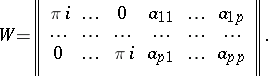The Riemann relations between the elements of the matrix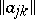,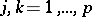, now ensure the symmetry of the matrix,, and the positive definiteness of the matrix of real parts,. However, for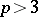there will be only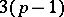independent elements among the elementsof, i.e. as many as the number of conformal moduli for the Riemann surfaceon which the inversion problem is solved (cf. Moduli of a Riemann surface). In addition to the Riemann relations there are in such a case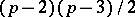transcendental relations between the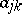, the explicit form of which for the case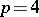was first found by E. Schottky in 1886; for a review of subsequent advances in the field see .

Special Abelian functions can be represented as the quotient of two entire theta-functions with half-integral characteristics of a special kind. These representations yield a number of properties of special Abelian functions which generalize many properties of elliptic functions. Thus, derivatives of an Abelian functionwith respect to any argument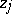are Abelian functions; any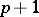Abelian functions are related by some algebraic equation; any Abelian function can be rationally expressed in terms of someAbelian functions, e.g. by means of an arbitrary Abelian function and itspartial derivatives of the first order; Abelian functions satisfy the addition theorem, i.e. the value of an Abelian function at the point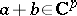may be rationally expressed in terms of the values of a certain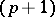-tuple of Abelian functions at the points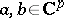.

Abelian functions are very important in algebraic geometry as a means of uniformization of algebraic varieties of certain types.